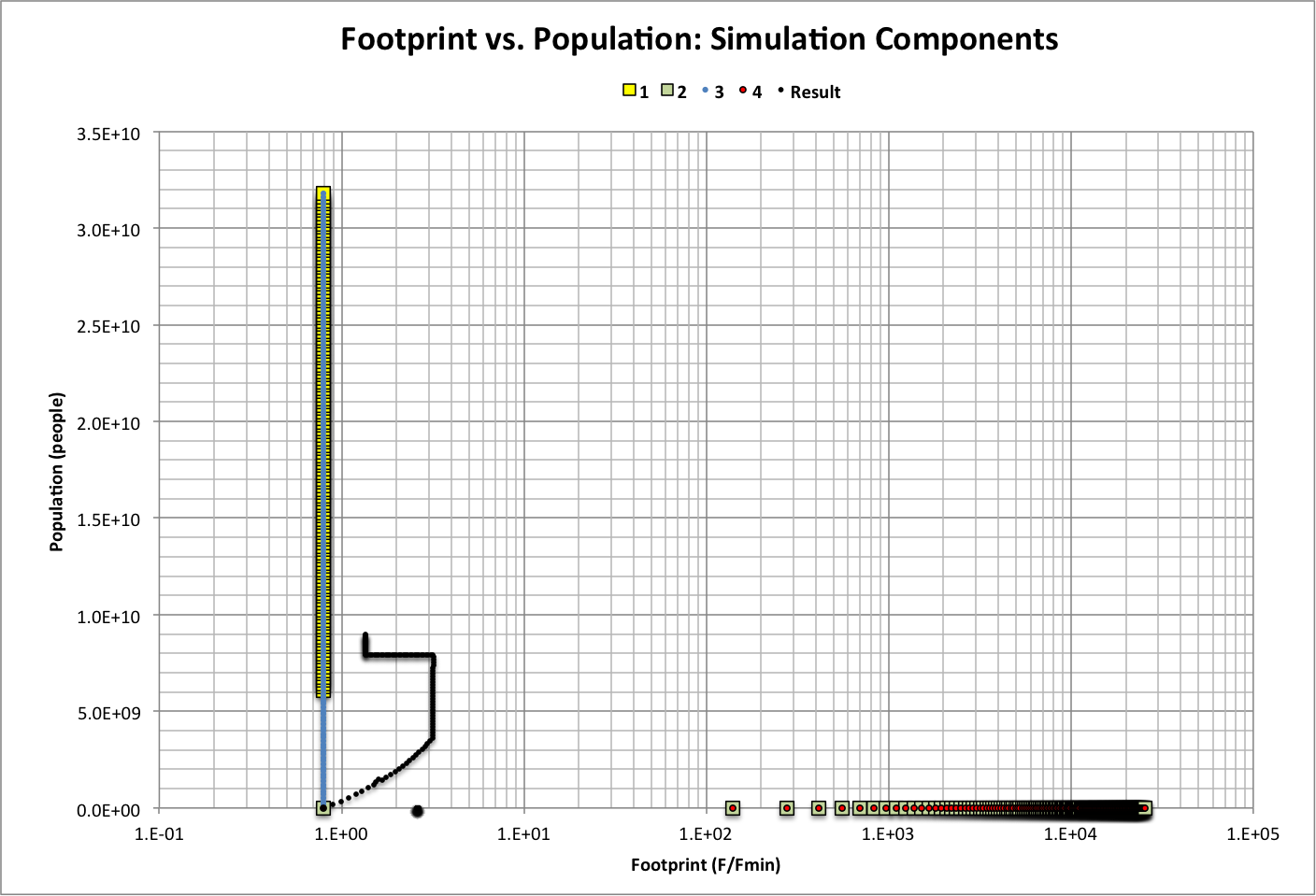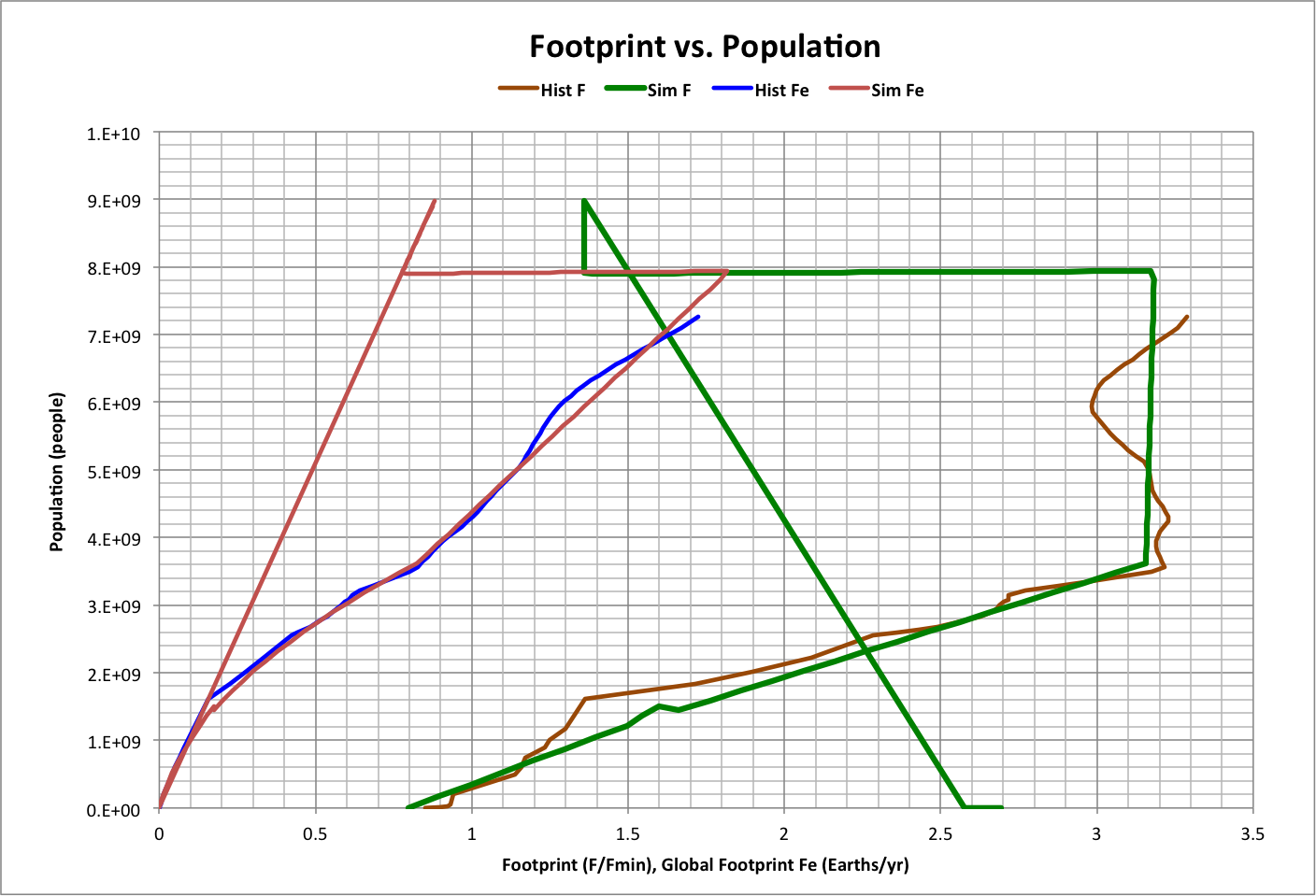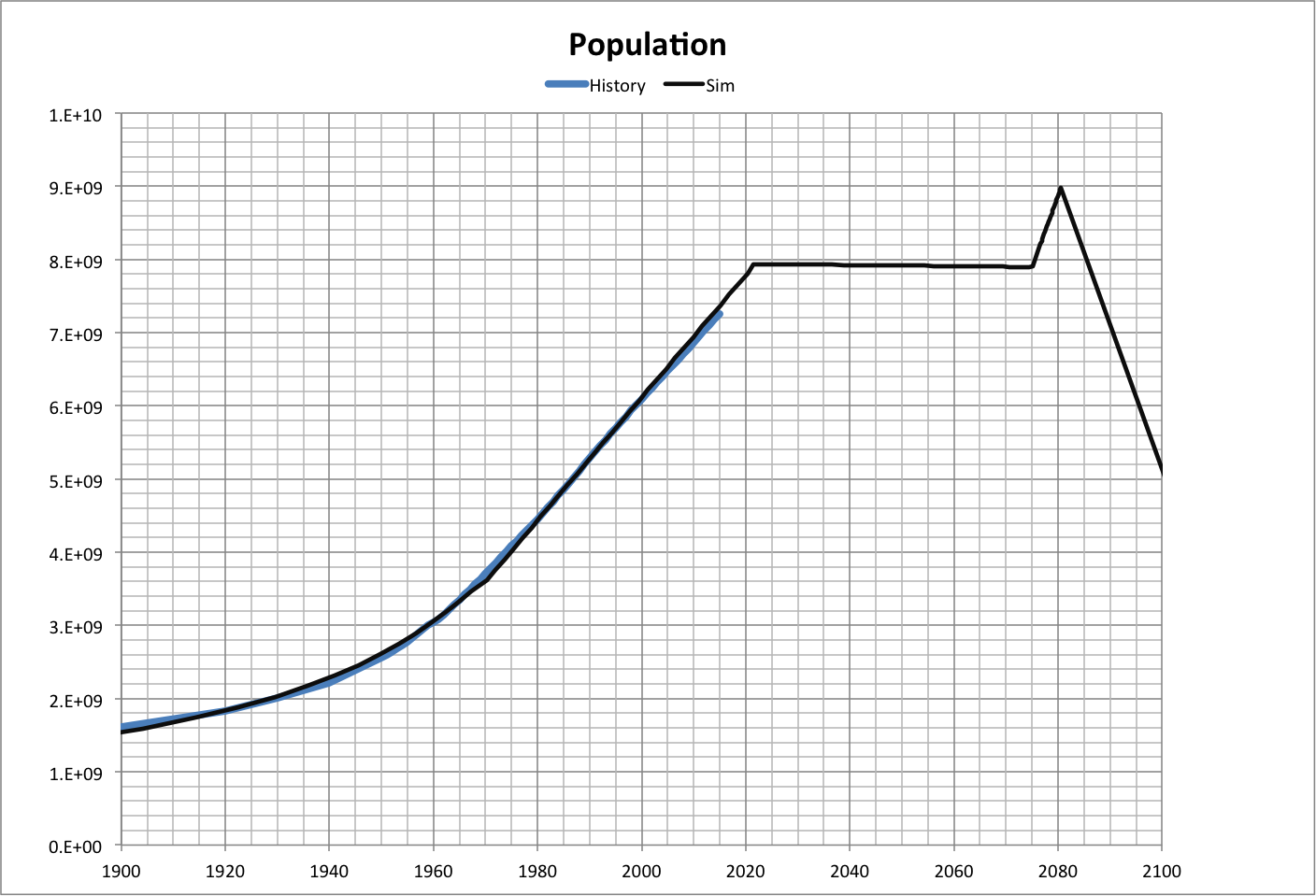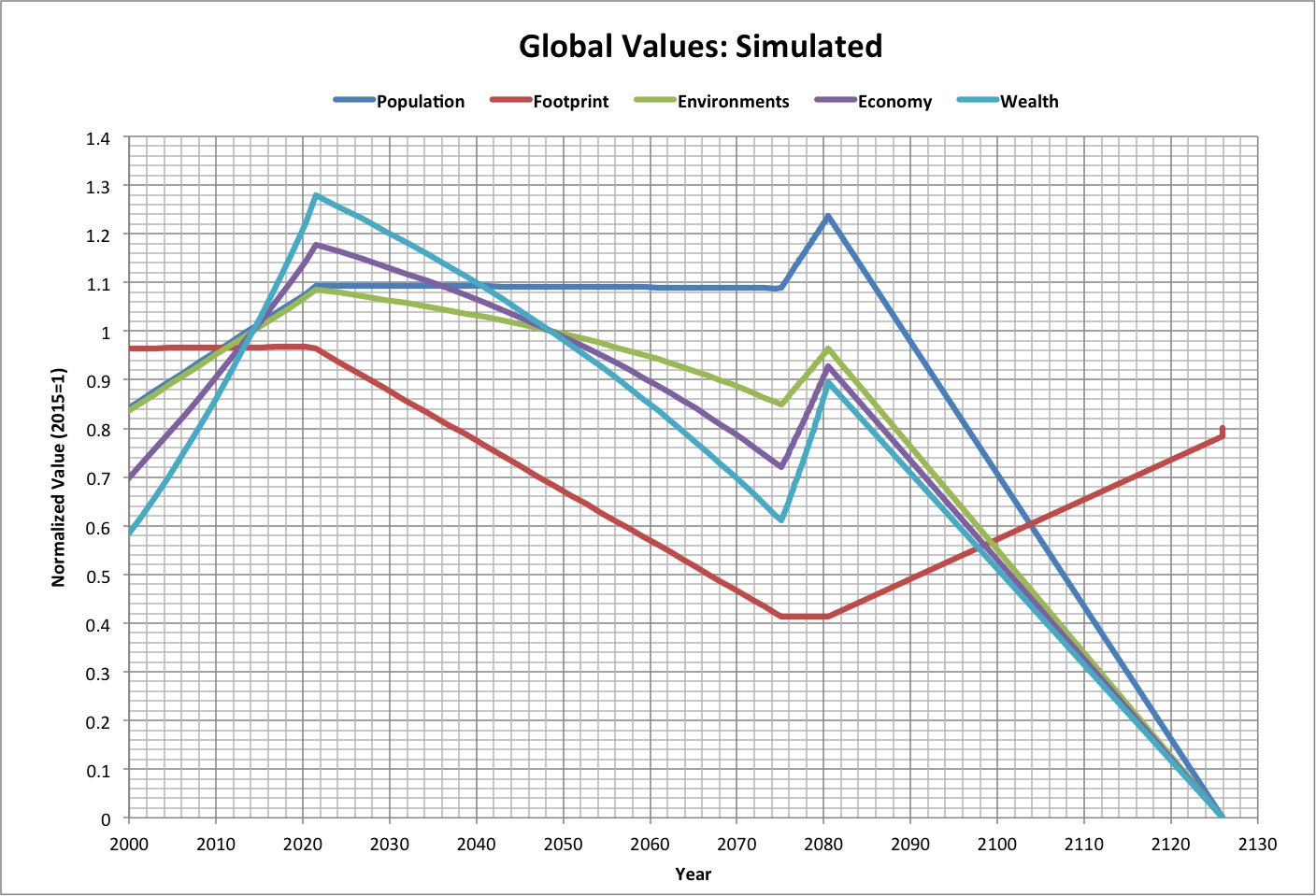# Backcast Version 2

Basic behavior of past population and consumption can be reproduced by adding the results of four simulations, each graphed below along with the result of combining them. Roll over image for a log-log plot.Here, F is the average per-capita ecological footprint in Earths/person/year and Fmin is the minimum ecological footprint. Points on the graph represent a value of global footprint (Fe = P * F, where P is population), separated in each simulation by a difference of 0.01 Earths/year. Simulations and the result all start at year 10000 B.C.E., when P = 1E+06 people and f0 = F0 / Fmin = 7.97E-01.

The simulations are calculated as follows:

Simulation 1

f1 = f0

P1 = Fe / (f1 * Fmin)

Maximum Limits:

 f1 (Felow / Fmin) / P1 - 2 P1 (Felow / Fmin) / (f1 + 2) Fe Fehigh
Simulation 2

f2 = (Fe / P2) / Fmin

P2 = P0

Maximum Limits:

 f2 (Felow / Fmin) / P2 - 2 P2 (Felow / Fmin) / (f2 + 2) Fe Fehigh
Simulation 3

f3 = f0

P3 = Fe / (f3 * Fmin)

Maximum Limits:

 f3 (Fhigh / Fmin) / P3 - 2 P3 (Fhigh / Fmin) / (f3 + 2) Fe Fehigh
Simulation 4

f4 = (Fe / P2) / Fmin

P4 = P0

Maximum Limits:

 f4 (Fhigh / Fmin) / P4 - 2 P4 (Fhigh / Fmin) / (f4 + 2) Fe Fehigh

The result is calculated using two equations:

Presult = (a1 * P1 + a2 * P2 + a3 * P3 + a4 * P4) * (fresult > 1)

+ (a1 * P1 + a2 * P2 + a3 * P3 + a4 * P4) *  fresult * (fresult <= 1)

fresult = a1 * f1 + a2 * f2 + a3 * f3 + a4 * f4

The weights ( an ) and maximum global footprints (Felow and Fehigh) are chosen to best fit the result to historical data:

a1 = 1.66546503E-01

a2 = 7.14484E-04

a3 = 8.32732517E-01

a4 = 6.49531E-06

Felow = 2.5E-01

Fehigh = 2.972069653

The resulting values of f , P, and Fe are shown below along with historical data and includes a futurecast with no global warming:Time t in years between two values of Fe can be estimated using the following relationships:

 Fe < 7.67E-01 Year < 1967 Pt = Pt-1 * (1 + Popratet)t Popratet = -0.0204562252684025 * Fet^2 + 0.0377807174089065 * Fet + 0.00141394695249756 Year = Year(P) = t - 2008 Year(Fe) >= 1967 Year(Fe) < = 2006 tn - tn-1  = (Feration - Feration-1) / (0.01 * Fen) Feratio = Fe / Fehigh Year(Fe) = t + 1797 Year(Fe) = Year(P) YearsFen = (tn - tn-1) / ( Fen - Fen-1) AVERAGE(YearsFe) = 52 Year > 1967 Yearn - Yearn-1 = AVERAGE(YearsFe) * ABS( Fen - Fen-1 ) where ABS is the absolute value

The validity of this estimate can be seen in the following graph of population. Roll over the image for a view of all population values.Normalizing global variables, the future is projected as follows (again, with no global warming). Roll over the image to also see historical values.